GED Math : Complementary Angles

Example Questions

1 2 3 4 6 Next →

Example Question #481 : 2 Dimensional Geometry

Find the the measure of angle B if it is complement of angle A: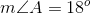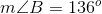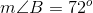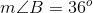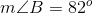Explanation:

If two angles are complementary, that means the sum of their degrees of measure will add up to 90. In order to find the measure of angle B, subtract angle A from 90 like shown: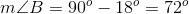This gives us a final answer of 72 degrees for angle B.

Example Question #482 : 2 Dimensional Geometry

Find the the measure of angle B if it is complement of angle A: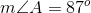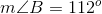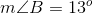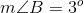Explanation:

If two angles are complementary, that means the sum of their degrees of measure will add up to 90. In order to find the measure of angle B, subtract angle A from 90 like shown: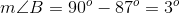This gives us a final answer of 3 degrees for angle B.

Example Question #483 : 2 Dimensional Geometry

Find the the measure of angle B if it is complement of angle A: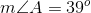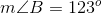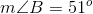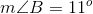Explanation:

If two angles are complementary, that means the sum of their degrees of measure will add up to 90. In order to find the measure of angle B, subtract angle A from 90 like shown: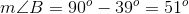This gives us a final answer of 51 degrees for angle B.

Example Question #484 : 2 Dimensional Geometry

Find the the measure of angle B if it is complement of angle A: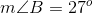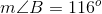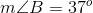Explanation:

If two angles are complementary, that means the sum of their degrees of measure will add up to 90. In order to find the measure of angle B, subtract angle A from 90 like shown: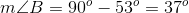This gives us a final answer of 37 degrees for angle B.

Example Question #485 : 2 Dimensional Geometry

Find the the measure of angle B if it is complement of angle A: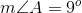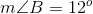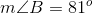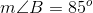Explanation:

If two angles are complementary, that means the sum of their degrees of measure will add up to 90. In order to find the measure of angle B, subtract angle A from 90 like shown: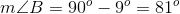This gives us a final answer of 81 degrees for angle B.

Example Question #486 : 2 Dimensional Geometry

If an angle measures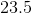degrees, find the measurement of the other angle such that the two angles are complementary.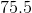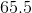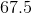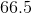Explanation:

Two angles are classified as complementary if an only if the sum of the angles equals to exactly 90 degrees.

Since we know the measurement of one angle, and we know the rule about complementary angles, let's find the other angle:

The other angle is simply the total sum of both angles minus the given angle.
The total sum of the angles is, and the given angle is.

So, we will subtractfrom.

Other angle=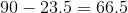The other angle has a measurement ofdegrees.

1 2 3 4 6 Next →